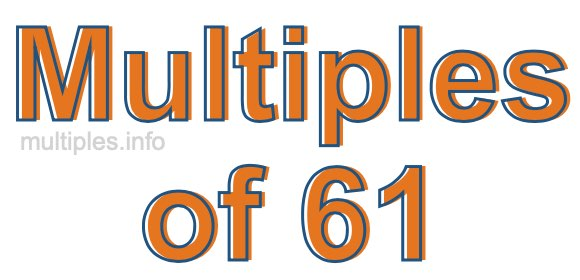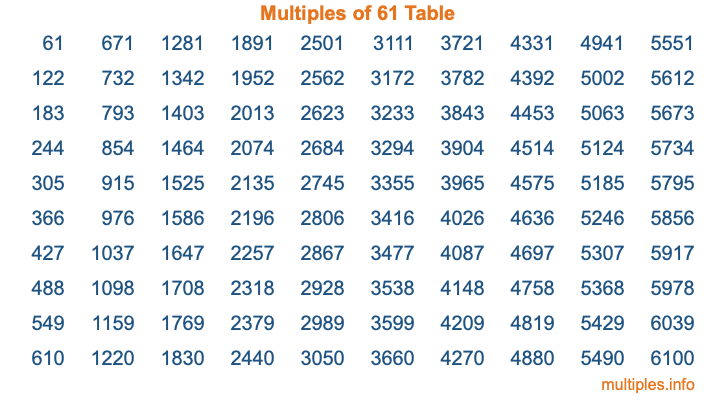Multiples of 61Welcome to the Multiples of 61 page. Here we will first teach you everything you will ever need to know about the multiples of 61, and then give you a study guide summary of everything we taught you to make sure you remember it all. Use this page to look up facts and learn information about the multiples of 61. This page will make you a multiples of sixty-one expert!

Definition of Multiples of 61
Multiples of 61 are all the numbers that when divided by 61 equal an integer. Each of the multiples of 61 are called a multiple. A multiple of 61 is created by multiplying 61 by an integer.

Therefore, to create a list of multiples of 61, you start with 1 multiplied by 61, then 2 multiplied by 61, then 3 multiplied by 61, and so on for as long as you want. Thus, the list of the first five multiples of 61 is 61, 122, 183, 244, and 305. To see a larger list of multiples of 61, see the printable image of Multiples of 61 further down on this page. We also have a category where you can choose any nth multiple of 61.

Multiples of 61 Checker
The Multiples of 61 Checker below checks to see if any number of your choice is a multiple of 61. In other words, it checks to see if there is any number (integer) that when multiplied by 61 will equal your number. To do that, we divide your number by 61. If the the quotient is an integer, then your number is a multiple of 61.

Is  a multiple of 61?

Least Common Multiple of 61 and ...
A Least Common Multiple (LCM) is the lowest multiple that two or more numbers have in common. This is also called the smallest common multiple or lowest common multiple and is useful to know when you are adding our subtracting fractions. Enter one or more numbers below (61 is already entered) to find the LCM.

Check out our LCM Calculator if you need more details about the Least Common Multiple or if you need the LCM for different numbers for adding and subtraction fractions.

nth Multiple of 61
As we stated above, 61 is the first multiple of 61, 122 is the second multiple of 61, 183 is the third multiple of 61, and so on. Enter a number below to find the nth multiple of 61.

th multiple of 61

Multiples of 61 vs Factors of 61
61 is a multiple of 61 and a factor of 61, but that is where the similarities end. All postive multiples of 61 are 61 or greater than 61. All positive factors of 61 are 61 or less than 61.

Below is the beginning list of multiples of 61 and the factors of 61 so you can compare:

Multiples of 61: 61, 122, 183, 244, 305, etc.

Factors of 61: 1, 61

As you can see, the multiples of 61 are all the numbers that you can divide by 61 to get a whole number. The factors of 61, on the other hand, are all the whole numbers that you can multiply by another whole number to get 61.

It's also interesting to note that if a number (x) is a factor of 61, then 61 will also be a multiple of that number (x).

Multiples of 61 vs Divisors of 61
The divisors of 61 are all the integers that 61 can be divided by evenly. Below is a list of the divisors of 61.

Divisors of 61: 1, 61

The interesting thing to note here is that if you take any multiple of 61 and divide it by a divisor of 61, you will see that the quotient is an integer.

Multiples of 61 Table
Below is an image of the first 100 multiples of 61 in a table. The table is in chronological order, column by column. The first column has the first ten multiples of 61, the second column has the next ten multiples of 61, and so on.The Multiples of 61 Table is also referred to as the 61 Times Table or Times Table of 61. You are welcome to print out our table for your studies.

Negative Multiples of 61
Although not often discussed or needed in math, it is worth mentioning that you can make a list of negative multiples of 61 by multiplying 61 by -1, then by -2, then by -3, and so on, to get the following list of negative multiples of 61:

-61, -122, -183, -244, -305, etc.

Multiples of 61 Summary
Below is a summary of important Multiples of 61 facts that we have discussed on this page. To retain the knowledge on this page, we recommend that you read through the summary and explain to yourself or a study partner why they hold true.

There are an infinite number of multiples of 61.

A multiple of 61 divided by 61 will equal a whole number.

61 divided by a factor of 61 equals a divisor of 61.

The nth multiple of 61 is n times 61.

The largest factor of 61 is equal to the first positive multiple of 61.

61 is a multiple of every factor of 61.

61 is a multiple of 61.

A multiple of 61 divided by a divisor of 61 equals an integer.

61 divided by a divisor of 61 equals a factor of 61.

Any integer times 61 will equal a multiple of 61.

Multiples of a Number
Here you can get the multiples of another number, all with the same attention to detail as we did for multiples of 61 on this page.

Multiples of
Multiples of 62
Did you find our page about multiples of sixty-one educational? Do you want more knowledge? Check out the multiples of the next number on our list!